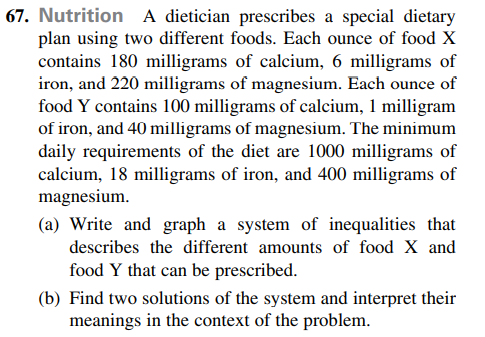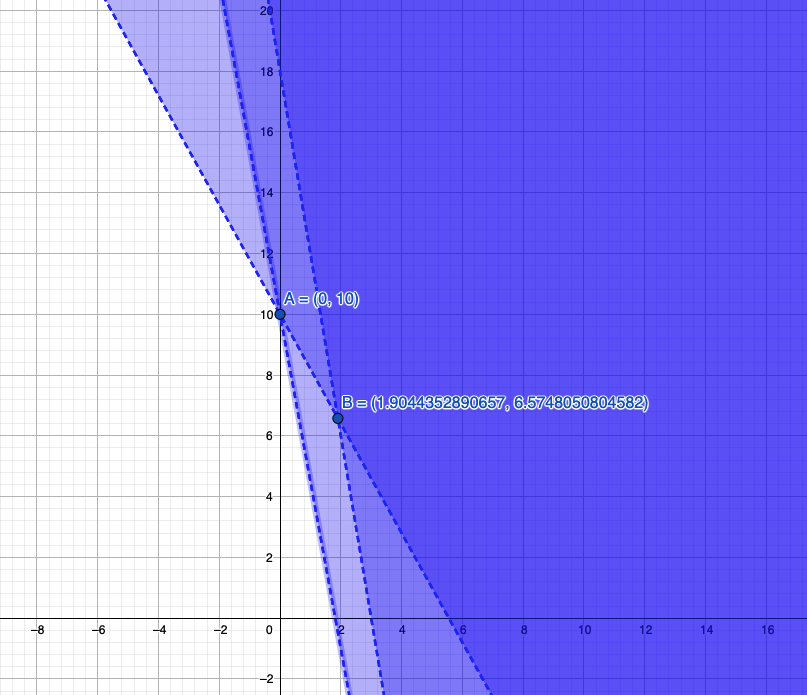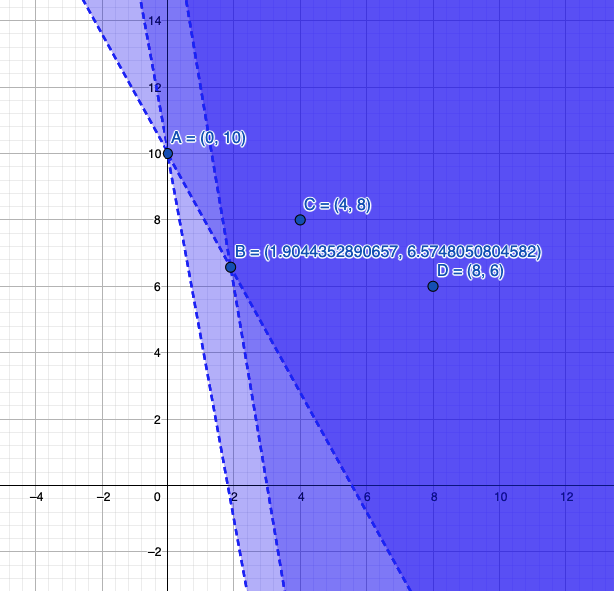### ¿Todavía tienes preguntas de matemáticas?

Pregunte a nuestros tutores expertos
Algebra
Pregunta

A dietician prescribes a special dietary plan using two different foods. Each ounce of food $$X$$ contains $$180$$ milligrams of calcium, $$6$$ milligrams of iron, and $$220$$ milligrams of magnesium. Each ounce of food $$Y$$ contains $$100$$ milligrams of calcium, $$1$$ milligram of iron, and $$40$$ milligrams of magnesium. The minimum daily requirements of the diet are $$1000$$ milligrams of calcium, $$18$$ milligrams of iron, and $$400$$ milligrams of magnesium.

(a) Write and graph a system of inequalities that describes the different amounts of food X and food $$Y$$ that can be prescribed.

(b) Find two solutions of the system and interpret their meanings in the context of the problem.a)

180x + 100y > 1000

6x + y > 18

220x + 40y > 400b)i) x = 4, y = 8

Consuming food X in quantity 4 ounces and  food Y in quantity 8 ounces, will fulfil the dietary requirements.

ii) x = 8, y = 6

Consuming food X in quantity 8 ounces and  food Y in quantity 6 ounces, will fulfil the dietary requirements.

Solución
View full explanation on CameraMath App.